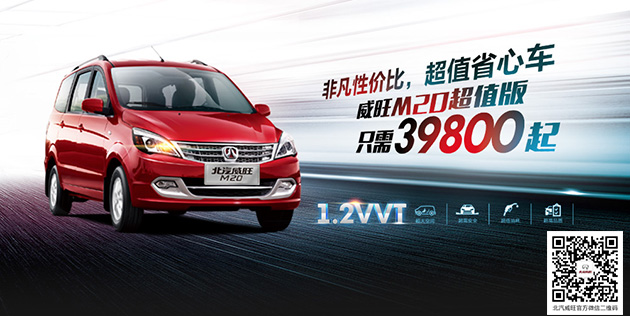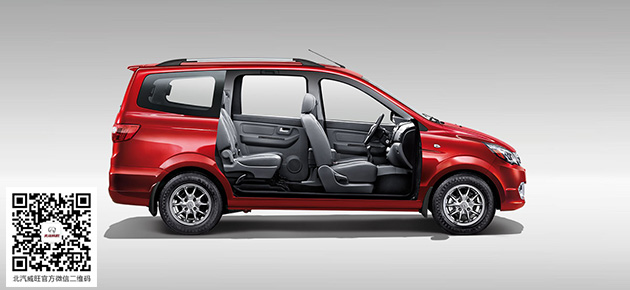## 大招抄底约“惠”忙 威旺M20车主超值购车记

2015年08月27日 08:46 来源：www.chextx.com 超过：次关注

前不久，在北京经营着一家粮油铺的赵先生计划购买一辆经济耐用的MPV车型，用以满足日常家用和生意上的商用需求。作为一个生意人，赵先生有着自己“固执”的选车标准，不仅要求价廉质优，且后期用车也要省心省钱，在反复对比后，赵先生购买了北汽威旺M20超值版车型。小编记录下了赵先生分享的独家购车攻略，希望能够为选车犹豫不决的朋友们，送上一颗购车“定心丸”。底价抢购 购车攻略倾囊相授

“购车时，你首先得明白你的用车需求是什么”，赵先生在采访的开始便如是说道。赵先生是两个孩子的爸爸，平日里接送孩子上下学，闲暇时间一家人也时常自驾出游，购车对日常家用的需求十分突出；正在经营的粮油铺日常进货、送货也少不了爱车帮忙，商用需求也必不可少。因此，赵先生一开始便将购车范围锁定在了“宜家宜商”的MPV之中。

购车之前，赵先生逛车主论坛、去4S店试驾，货比三家之后，最终在8月初购入了威旺M20超值版。赵先生说，从选车时开始，威旺M20的促销力度便深深地吸引了他，买车优惠还送保养，大大降低了后期用车成本。据了解，8月份，威旺M20全系直降3000元，购入威旺M20超值版仅需37800元，同时赠送价值2000元的2年8次免费保养，单车优惠额度超过5000元。如此诱人的价格是最终促成赵先生购车的重要因素，低价质优、贴心服务——威旺M20给了精明的赵先生一颗大大的“定心丸”。

价廉质优 经济耐用生意无忧

除了诱人的购车底价，促使赵先生购车的主要因素是威旺M20超值版的高性价比。据赵先生介绍，当初在购车时也深入地了解过五菱宏光、长安欧诺等热销车型，然而在多方对比后，赵先生还是选择了威旺M20超值版，因为该车不仅价格超值，品质也十分出众。赵先生说，在几款车车内空间的对比上，威旺M20超值版2790mm的超长轴距保证了车内空间的灵活多变，平时载人载货都显得游刃有余，车内空间是他特别满意的地方。在安全性能上，威旺M20超值版的高强度车身安全结构设计对车身进行了全面强化，使一家老小的乘车安全更有保证。

不仅如此，威旺M20超值版搭载的1.2L发动机及采用的先进CVVT和EGR环保节油技术有效地控制了油耗，6.5L/100Km的超低油耗大大节省了平日里的用车成本。凭借着空间、配置、节油等各方面优势，威旺M20超值版短时间内便成为了赵先生生活中的好伙伴，生意上的好助手。

威旺M20自上市以来，便以卓越的品质赢取了众多消费者的心，赵先生购入威旺M20仅半个月，也称其是紧凑型MPV界当之无愧的“国民车”。这个8月，北汽威旺诚意载钜惠，给予消费者人人可享的真正实惠，诚意回馈力度空前，限时特惠，别再犹豫！

#### 相关文章

﻿
• 快速找车
• 选择品牌
• 选择品牌
• A  奥迪
• A  阿斯顿·马丁
• A  阿尔法·罗密欧
• B  宝沃
• B  布加迪
• B  巴博斯
• B  保时捷
• B  宾利
• B  奔驰
• B  宝马
• B  本田
• B  别克
• B  标致
• B  比亚迪
• B  宝骏
• B  北汽制造
• B  北汽新能源
• B  北汽幻速
• B  北汽威旺
• B  北京汽车
• B  奔腾
• B  北汽绅宝
• B  北汽昌河
• C  长安欧尚
• C  长安
• C  长安凯程
• C  长城
• D  大众
• D  道奇
• D  DS
• D  东南
• D  东风风神
• D  东风风行
• D  东风小康
• D  东风风度
• D  东风
• F  福特
• F  丰田
• F  菲亚特
• F  法拉利
• F  福田
• F  福迪
• F  福汽启腾
• G  观致
• G  广汽传祺
• G  广汽吉奥
• G  GMC
• H  红旗
• H  汉腾汽车
• H  哈弗
• H  哈飞
• H  海格
• H  海马
• H  华颂
• H  黄海
• H  华泰
• H  恒天
• J  几何汽车
• J  捷达
• J  吉利汽车
• J  捷豹
• J  Jeep
• J  江淮
• J  江铃
• J  金杯
• J  九龙
• J  金旅
• K  凯翼
• K  凯迪拉克
• K  克莱斯勒
• K  科尼塞克
• K  卡威
• K  开瑞
• L  路虎
• L  林肯
• L  劳斯莱斯
• L  兰博基尼
• L  雷克萨斯
• L  铃木
• L  领克
• L  雷诺
• L  理念
• L  力帆
• L  莲花汽车
• L  猎豹
• L  路特斯
• L  陆风
• M  马自达
• M  MG
• M  MINI
• M  玛莎拉蒂
• M  摩根
• M  迈凯轮
• N  纳智捷
• O  欧拉
• O  欧宝
• O  讴歌
• O  欧朗
• Q  奇瑞
• Q  起亚
• Q  启辰
• R  日产
• R  荣威
• R  瑞麒
• S  SERES赛力斯
• S  三菱
• S  斯威汽车
• S  萨博
• S  smart
• S  斯柯达
• S  斯巴鲁
• S  思铭
• S  双龙
• S  上汽大通
• S  双环
• T  特斯拉
• T  腾势
• W  蔚来
• W  沃尔沃
• W  WEY
• W  五菱汽车
• W  五十铃
• W  威兹曼
• W  威麟
• X  现代
• X  雪佛兰
• X  星途
• X  雪铁龙
• X  小鹏汽车
• X  西雅特
• Y  一汽
• Y  英菲尼迪
• Y  英致
• Y  依维柯
• Y  野马汽车
• Y  永源
• Z  众泰
• Z  中华
• Z  中兴
• Z  知豆
• 选择车系
• 选择车系
• 车型对比
• 选择品牌
• 选择品牌
• A  奥迪
• A  阿斯顿·马丁
• A  阿尔法·罗密欧
• B  宝沃
• B  布加迪
• B  巴博斯
• B  保时捷
• B  宾利
• B  奔驰
• B  宝马
• B  本田
• B  别克
• B  标致
• B  比亚迪
• B  宝骏
• B  北汽制造
• B  北汽新能源
• B  北汽幻速
• B  北汽威旺
• B  北京汽车
• B  奔腾
• B  北汽绅宝
• B  北汽昌河
• C  长安欧尚
• C  长安
• C  长安凯程
• C  长城
• D  大众
• D  道奇
• D  DS
• D  东南
• D  东风风神
• D  东风风行
• D  东风小康
• D  东风风度
• D  东风
• F  福特
• F  丰田
• F  菲亚特
• F  法拉利
• F  福田
• F  福迪
• F  福汽启腾
• G  观致
• G  广汽传祺
• G  广汽吉奥
• G  GMC
• H  红旗
• H  汉腾汽车
• H  哈弗
• H  哈飞
• H  海格
• H  海马
• H  华颂
• H  黄海
• H  华泰
• H  恒天
• J  几何汽车
• J  捷达
• J  吉利汽车
• J  捷豹
• J  Jeep
• J  江淮
• J  江铃
• J  金杯
• J  九龙
• J  金旅
• K  凯翼
• K  凯迪拉克
• K  克莱斯勒
• K  科尼塞克
• K  卡威
• K  开瑞
• L  路虎
• L  林肯
• L  劳斯莱斯
• L  兰博基尼
• L  雷克萨斯
• L  铃木
• L  领克
• L  雷诺
• L  理念
• L  力帆
• L  莲花汽车
• L  猎豹
• L  路特斯
• L  陆风
• M  马自达
• M  MG
• M  MINI
• M  玛莎拉蒂
• M  摩根
• M  迈凯轮
• N  纳智捷
• O  欧拉
• O  欧宝
• O  讴歌
• O  欧朗
• Q  奇瑞
• Q  起亚
• Q  启辰
• R  日产
• R  荣威
• R  瑞麒
• S  SERES赛力斯
• S  三菱
• S  斯威汽车
• S  萨博
• S  smart
• S  斯柯达
• S  斯巴鲁
• S  思铭
• S  双龙
• S  上汽大通
• S  双环
• T  特斯拉
• T  腾势
• W  蔚来
• W  沃尔沃
• W  WEY
• W  五菱汽车
• W  五十铃
• W  威兹曼
• W  威麟
• X  现代
• X  雪佛兰
• X  星途
• X  雪铁龙
• X  小鹏汽车
• X  西雅特
• Y  一汽
• Y  英菲尼迪
• Y  英致
• Y  依维柯
• Y  野马汽车
• Y  永源
• Z  众泰
• Z  中华
• Z  中兴
• Z  知豆
• 选择车系
• 选择车系
• 选择车型
• 选择车型
• 意见反馈